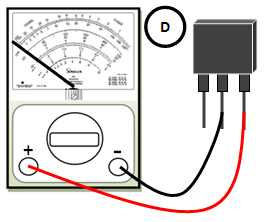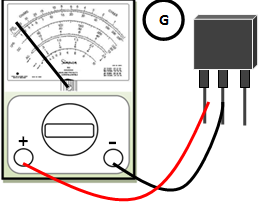## Identifying the electrodes and type of bipolar transistor.

Note: Range x1 or x10 is used in illustrations A to F.
1. Connect the test probes to the terminal of the transistor.Observe that the pointer needle did not deflect. Then reverse the connection as shown on illustration B.
2. In this illustration, the pointer needle deflects to the right. In this case, we can say that the first or the second terminal is the base.
3. Move the test probe from first terminal to the third terminal. In the illustration the pointer needle deflects to the right and this means that the center terminal is the base and the type of transistor is PNP.
4. In this illustration, the pointer needle did not deflect which proves that the center terminal is the base and a PNP.
5.  It is done to test that there is no deflection between the first and last terminal. If the pointer needle deflects, the bipolar transistor is shorted.
6.   It is done to test that there is no deflection between the first and last terminal. If the pointer needle deflects, the bipolar transistor is shorted.

Identifying the emitter and collector of bipolar transistor
7. To identify the emitter and collector, set the ohmmeter to Rx10k or equivalent. Apply reverse bias to the electrodes of transistor, since the transistor is PNP, connect the black test probe to the center terminal (base) and the red test probe to the first terminal.
8. On the illustration above, the red test probe is moved to the last terminal while the black test probe is left to the center terminal. Compare the meter deflections, illustration G shows higher resistance compared to illustration H. The one with the lower resistance is the emitter while the one with high resistance is the collector.Connect the test probes to the terminal of the transistor.Observe that the pointer needle did not deflect. Then reverse the connection as shown on illustration B.In this illustration, the pointer needle deflects to the right. In this case, we can say that the first or the second terminal is the base.Move the test probe from first terminal to the third terminal. In the illustration the pointer needle deflects to the right and this means that the center terminal is the base and the type of transistor is PNP.In this illustration, the pointer needle did not deflect which proves that the center terminal is the base and a PNP.It is done to test that there is no deflection between the first and last terminal. If the pointer needle deflects, the bipolar transistor is shorted.It is done to test that there is no deflection between the first and last terminal. If the pointer needle deflects, the bipolar transistor is shorted.To identify the emitter and collector, set the ohmmeter to Rx10k or equivalent. Apply reverse bias to the electrodes of transistor, since the transistor is PNP, connect the black test probe to the center terminal (base) and the red test probe to the first terminal.The red test probe is moved to the last terminal while the black test probe is left to the center terminal. Compare the meter deflections, illustration G shows higher resistance compared to illustration H. The one with the lower resistance is the emitter while the one with high resistance is the collector.